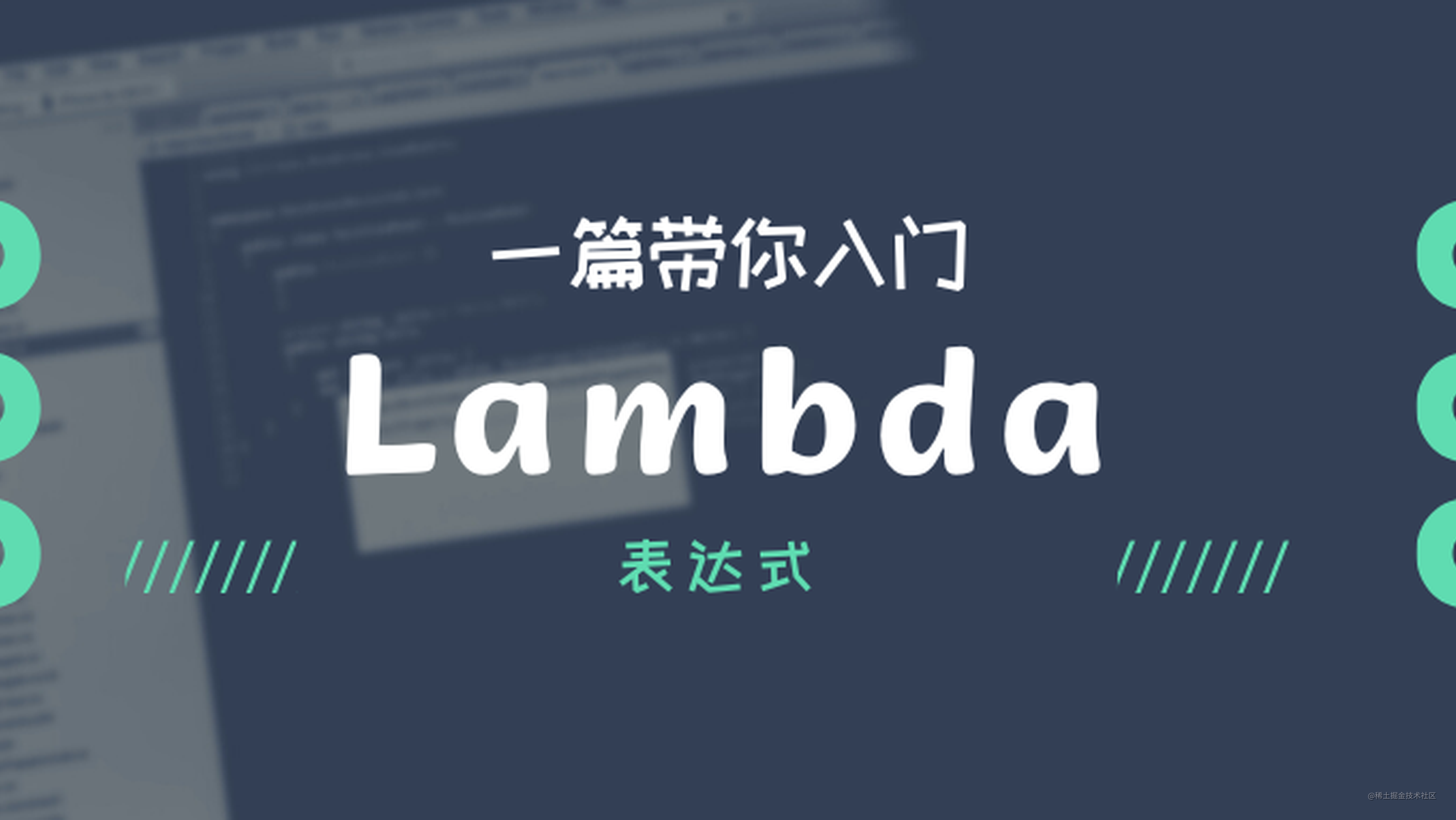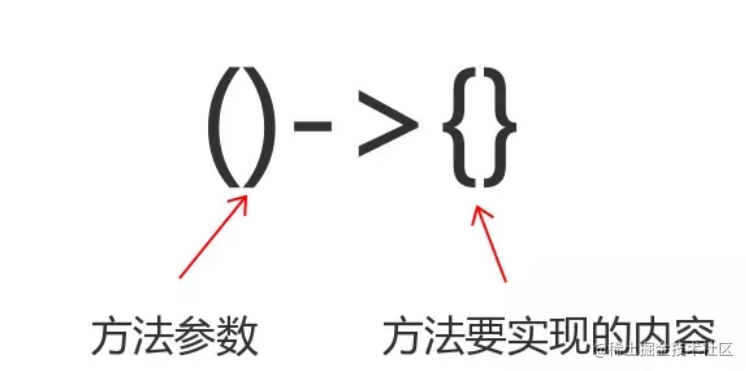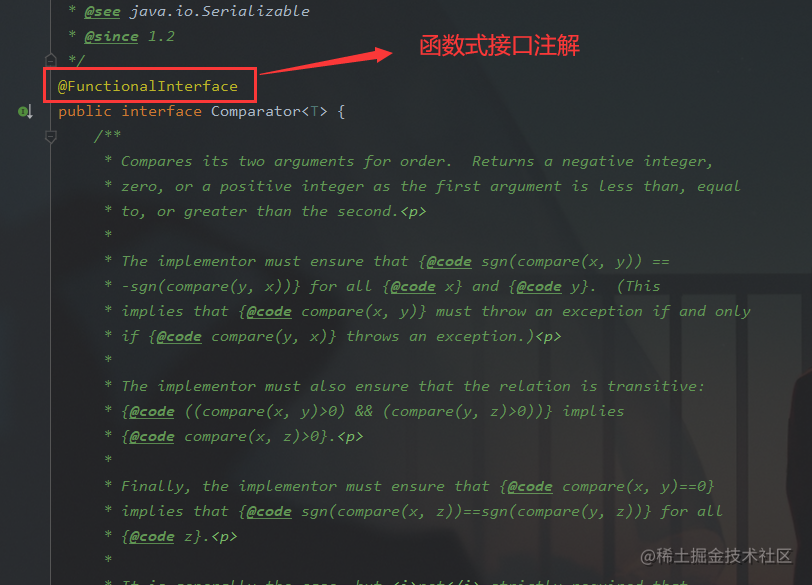# Lambda 表达式## Lambda

Lambda 是一个匿名函数，可以把 Lambda 表达式理解为是一段可以传递的代码（将代码像数据一样进行传递）。使用它可以写出更简洁、更灵活的代码。作为一种更紧凑的代码风格，使 Java 的语言表达能力得到了提升。• Lambda 表达式的语法显然比匿名内部类更简洁：
``````// 匿名内部类
new Thread (new Runnable() {
@Override
public void run() {
System.out.println("One man's constant is another man's variable.");
}
});

// Lambda 表达式
new Thread(()-> System.out.println("One man's constant is another man's variable.")).start();

### Lambda 表达式的其他形式：

• Lambda 需要一个参数，但无返回值
``````// 接口需要一个形参
Consumer<String> consumer = (String s)  -> {
System.out.println(s);
};

consumer.accept("One man's constant is another man's variable.");

• 类型推断，可省略形参的数据类型
``````// 省略参数类型声明 - String
Consumer<String> consumer = (s) -> {
System.out.println(s);
};

consumer.accept("One man's constant is another man's variable.");

• 若 Lambda 表达式只需一个参数且仅有一条语句时`()``{}``return`(若有返回值) 可省略
``````// 接口仅需一个参数，可省略小括号
Consumer<String> consumer = s -> System.out.println(s);

consumer.accept("One man's constant is another man's variable.");

## 函数式编程接口### Java 内置四大核心函数式接口

void accept(T t)

T get()

R apply(T t)

boolean test(T t)

``````@Test
public void demo() {
List<String> list = Arrays.asList("北京", "南京", "天津");

List<String> filtered = filterString(list, s -> s.contains("京"));
System.out.println(filtered);
}

public List<String> filterString(List<String> list, Predicate<String> pre) {
// 过滤后的集合
ArrayList<String> filteredList = new ArrayList<>();
for (String s : list) {
if (pre.test(s)) {
}
}
return filterList;
}

## 方法引用

### 主要分为以下三种情况

• 对象 :: 实例方法
``````@Test
public void demo() {
Assassin w = new Assassin("伍六柒", 16);
// 调用 Assassin 对象 w 的 getName() 方法
Supplier<String> sup = w::getName;
System.out.println(sup.get());
}

• 类 :: 静态方法
``````@Test
public void demo() {
// 调用 Math 的 round() 方法
Function<Double, Long> func2 = Math::round;
System.out.println(func2.apply(12.6));
}

• 类 :: 实例方法
``````@Test
public void demo() {
BiPredicate<String, String> pre = String::equals;
// 调用 String 的 equals() 方法
System.out.println(pre.test("and", "or"));
}

## 构造器引用

``````// Lambda 表达式
Function<Integer, MyClass> lambda = (n)->new MyClass(n);

// 构造器引用
Function<Integer, MyClass> constructor = MyClass::new;

## 数组引用

``````// Lambda 表达式
Function<Integer, Integer[]> lambda = (n)->new Integer[n];

// 数组引用
Function<Integer, Integer[]> arr = (n)->Integer[]::new;